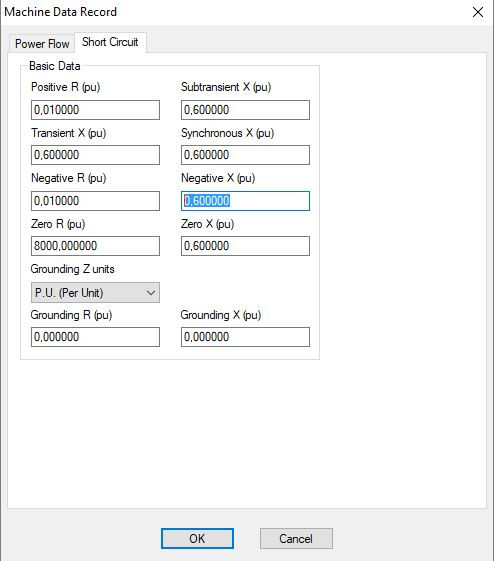Hi

Do you know how can I add negative sequence impedance (or admittance) for transmission line? Transformer? Generator? And load?

When I open each of them Branch, I can only see positive sequence and zero sequence, I can see where I can add the negative sequence impedance?

In transformer, I can only see the positive sequence impedance and I'm adding the zero sequence admittance to (X01), but I don't know where to add the negative sequence admittance?

In generator, I can't find where I can add the X2 values?

edit retag close merge delete

Sort by » oldest newest most votedFor passive elements like branches and transformers the negative sequence impedance is same as positive sequence impedance so there is no need to enter the values, Z2 = Z1. For generators there are data fields for negative R and negative X in the Machine tab in Network data spreadsheet. The Machine Data Record is shown below:more

Thank you so much! I also have to add negative G and B, it doesn't show it here? Do you know where is it? I only found the zero G and B?

Which component are you meaning? Shunts do not have negative sequence data fields in PSSE, Z2=Z1.

Great, thank you!!

more